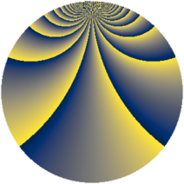# Properties

 Label 3630.2.bvLevel $3630$ Weight $2$ Character orbit 3630.bv Rep. character $\chi_{3630}(7,\cdot)$ Character field $\Q(\zeta_{220})$ Dimension $10560$ Sturm bound $1584$

# Related objects

## Defining parameters

 Level: $$N$$ $$=$$ $$3630 = 2 \cdot 3 \cdot 5 \cdot 11^{2}$$ Weight: $$k$$ $$=$$ $$2$$ Character orbit: $$[\chi]$$ $$=$$ 3630.bv (of order $$220$$ and degree $$80$$) Character conductor: $$\operatorname{cond}(\chi)$$ $$=$$ $$605$$ Character field: $$\Q(\zeta_{220})$$ Sturm bound: $$1584$$

## Dimensions

The following table gives the dimensions of various subspaces of $$M_{2}(3630, [\chi])$$.

Total New Old
Modular forms 64000 10560 53440
Cusp forms 62720 10560 52160
Eisenstein series 1280 0 1280

## Trace form

 $$10560q - 16q^{5} - 40q^{7} + O(q^{10})$$ $$10560q - 16q^{5} - 40q^{7} + 44q^{10} + 8q^{11} + 88q^{13} - 12q^{15} - 264q^{16} - 40q^{17} + 84q^{22} + 16q^{23} - 40q^{25} + 16q^{26} - 20q^{28} + 16q^{31} - 12q^{33} - 264q^{36} - 232q^{37} - 40q^{41} + 12q^{42} + 40q^{46} + 88q^{47} + 80q^{50} + 80q^{51} + 40q^{52} + 72q^{53} + 28q^{55} - 16q^{56} + 80q^{57} + 12q^{58} + 24q^{60} + 80q^{61} + 80q^{62} + 40q^{63} + 32q^{66} + 336q^{67} + 40q^{68} + 12q^{70} - 20q^{73} + 32q^{75} - 88q^{76} - 24q^{80} + 2640q^{81} + 32q^{82} + 192q^{85} + 32q^{86} - 4q^{88} + 40q^{91} + 72q^{92} - 24q^{93} + 40q^{95} + 96q^{97} + O(q^{100})$$

## Decomposition of $$S_{2}^{\mathrm{new}}(3630, [\chi])$$ into newform subspaces

The newforms in this space have not yet been added to the LMFDB.

## Decomposition of $$S_{2}^{\mathrm{old}}(3630, [\chi])$$ into lower level spaces

$$S_{2}^{\mathrm{old}}(3630, [\chi]) \cong$$ $$S_{2}^{\mathrm{new}}(605, [\chi])$$$$^{\oplus 4}$$$$\oplus$$$$S_{2}^{\mathrm{new}}(1210, [\chi])$$$$^{\oplus 2}$$$$\oplus$$$$S_{2}^{\mathrm{new}}(1815, [\chi])$$$$^{\oplus 2}$$